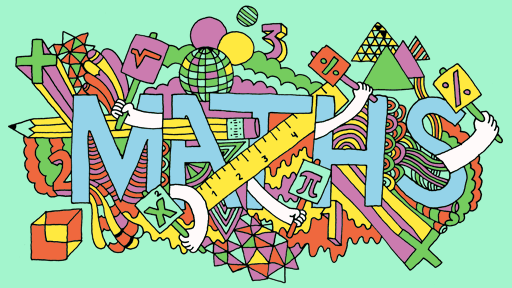Good morning Year 4!

Today, we have some factor pairs for you to have a look at.

Factor pairs are two numbers that multiply together to give you a product. In the example below, we look at factor pairs of 12. Any numbers that multiply together to make 12 gives you a factor pair (Follow this link to access the video) https://fb.watch/2SE8Rnapjr/

How many different factor pairs can you make for the following number:
– 24
– 36
– 48
– 64